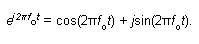?
EE Times-Asia > Processors/DSPs
?
?
Processors/DSPs??# Frequency domain tutorial: Understanding spectral components (Part II)

Posted: 04 Feb 2009 ?? ?Print Version ?

Keywords:components spectral? signal quadrature? tutorial domain?

Let's now focus on describing a quadrature signal, having a real and an imaginary part, that is a function time. To do so, we must remember that—as the great mathematician Karl Gauss first recommended—a single complex number can be represented by a point on the two-dimensional complex plane.

Such a plane has two axes (real and imaginary) that are orthogonal to each other, which means there is a 90° difference in the axes' orientations. Consider a complex number whose magnitude is one and whose phase angle increases with time. That complex number is the ej2πfot point shown on the complex plane in Figure 4. (Here the 2πfo term is frequency in radians/second corresponding to a frequency of fo cycles/second, where fo is measured in hertz.) As time t increases, the complex number's phase angle φ = 2πfot increases and our number orbits the origin of the complex plane in a counterclockwise direction.

Figure 4 shows that number, represented by the solid dot, frozen at some arbitrary instant in time. (That rotating ej2πfot complex number goes by two names in the DSP literature; it's often called a "complex exponential," and it's also referred to as a "quadrature signal.") If the frequency fo = 2Hz, the solid dot would rotate around the circle two times or two cycles per second.Figure 4: As time t increases, the complex number's phase angle φ = 2πfot increases and our number orbits the origin of the complex plane in a counterclockwise direction.

Because complex numbers can be represented in both polar and rectangular notation, we can represent our polar ej2πfot quadrature signal (using one of Leonhard Euler's identities) in rectangular form as:Equation 2

Equation 2 above tells us that as ej2πfotrotates around the origin, its real part, its East-West distance from the origin, varies as a cosine wave. The complex exponential's imaginary part, the North-South distance from the origin, varies as a sinewave. (Understanding the nature of a sinusoidal quadrature signal is no more difficult than reading a road map.) The attributes of our two-dimensional ej2πfot complex exponential are best illustrated with a 3D time-domain plot (Figure 5). Notice how the ej2πfot signal spirals so beautifully along the time axis with its real part being a cosine wave and its imaginary part being a sinewave. At time t = 0, the signal has a value of 1 + j 0 as we would expect. (Equation 2 allows us to represent a single complex exponential as the orthogonal sum of real cosine and real sine functions.)The attributes of our two-dimensional ej2πfot complex exponential are best illustrated with a 3D time-domain plot

That ej2πfot signal is not just mathematical mumbo jumbo. We can physically generate an ej2πfot signal and transmit it to a laboratory down the hall. All we need is two equal-amplitude sinusoidal signal generators, set to the same frequency fo. However, somehow we have to synchronize those two hardware generators so that their relative phase shift is fixed at 90°. Their outputs need to be orthogonal.)

Next, we connect coax cables to the generators' output connectors and run those two cables, labeled "Cosine" for our cosine signal and "Sine" for our sinewave signal, down the hall to their destination. In the other lab, if the continuous real signals were connected to the horizontal and vertical input channels of an oscilloscope (Figure 6), we'd see a bright spot rotating counterclockwise in a circle on the scope's display. (Remembering, of course, to set the scope's Horizontal Sweep control to the 'External' position.)Figure 6: Quadrature ej2πfot signal oscilloscope display

Pop quiz: What would be seen on the scope's display if the cables were mislabeled and the two real signals were inadvertently swapped? If you said we'd see another circle orbiting in a clockwise direction, pat yourself on the back because you'd be correct.

This oscilloscope example helps us answer the important question: "When we work with quadrature signals, how is the j?operator implemented in hardware?" The answer is that the j?operator is implemented by how we treat the two real signals relative to each other. We have to treat them orthogonally such that the cosine signal represents the Real (East-West) value, and the sinewave signal represents the Imaginary (North-South) value. So in our oscilloscope example the j?operator is implemented merely by how the connections are made to the scope, and the result is a two-dimensional quadrature signal represented by the instantaneous position of the dot on the scope's display.

By the way, if we control the instantaneous phase of the ej2πfot signal based on some bipolar binary data (+1 and -1), a person on the other lab could measure that phase at certain instants in time and extract that binary data. Many digital communications systems operate on this principle.

At this point you may ask, "Where does the idea of negative frequency come in here?" Well, there's a "Negative Frequency" signpost up ahead, and we're now ready to answer that question.

?
?
WebinarsVisit Asia Webinars to learn about the latest in technology and get practical design tips.

?
?